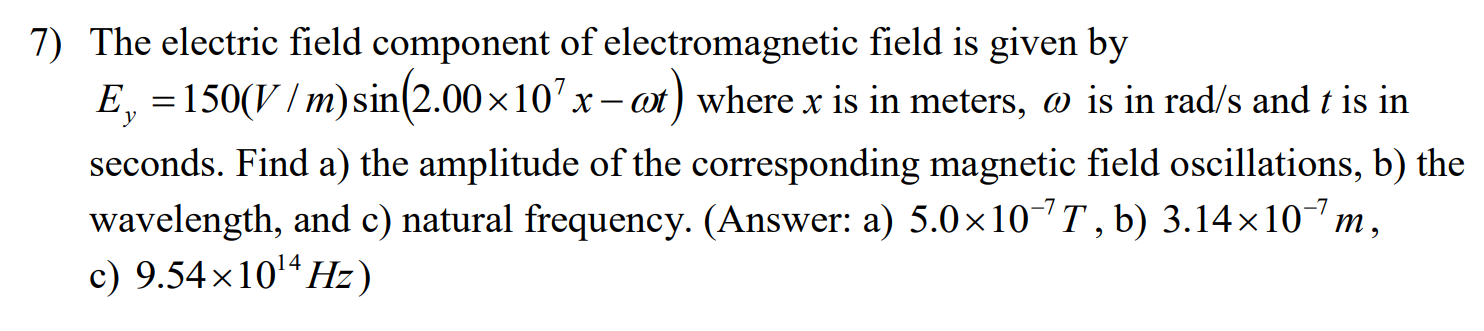# 7) The electric field component of electromagnetic field is given byE, 150(V/m)sin(2.00 x 10 :ot) where x is in meters, o is in rad/s and t is inхseconds. Find a) the amplitude of the corresponding magnetic field oscillations, b) thewavelength, and c) natural frequency. (Answer: a) 5.0 x 10 T, b) 3.14 x 107 mc) 9.54x1014Hz)X

Questionhelp_outlineImage Transcriptionclose7) The electric field component of electromagnetic field is given by E, 150(V/m)sin(2.00 x 10 : ot) where x is in meters, o is in rad/s and t is in х seconds. Find a) the amplitude of the corresponding magnetic field oscillations, b) the wavelength, and c) natural frequency. (Answer: a) 5.0 x 10 T, b) 3.14 x 107 m c) 9.54x1014Hz) X fullscreen
check_circleExpert Solution
Step 1

(a) Write the relation between amplitude of electric and magnetic field

Step 2

Substitute the values

Step 3

(b)
Write the expression f...

### Want to see the full answer?

See Solution

#### Want to see this answer and more?

Solutions are written by subject experts who are available 24/7. Questions are typically answered within 1 hour*

See Solution
*Response times may vary by subject and question
Tagged in

### Science